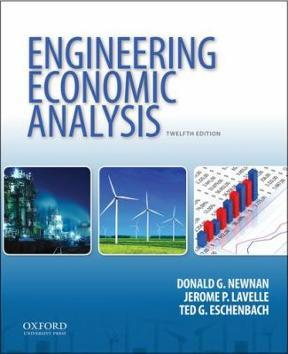×
×

# Modifying an assembly line has a first cost of\$80,000, andISBN: 9780199339273 93

## Solution for problem 10-17 Chapter 10

Engineering Economic Analysis | 12th Edition

• Textbook Solutions
• 2901 Step-by-step solutions solved by professors and subject experts
• Get 24/7 help from StudySoup virtual teaching assistantsEngineering Economic Analysis | 12th Edition

4 5 1 406 Reviews
26
4
Problem 10-17

Modifying an assembly line has a first cost of\$80,000, and its salvage value is \$0. The firmsinterest rate is 9%. The savings shown in the tabledepend on whether the assembly line runs one, two,or three shifts, and on whether the product is madefor 3 or 5 years.Shifts/ Savings/Useful Lifedayear Probability (years) Probability1 \$15,000 0.3 3 0.62 30,000 0.5 5 0.43 45,000 0.2 (a)Give the joint probability distribution for sav-ings per year and the useful life.(b)Define optimistic, most likely, and pessimisticscenarios by using both optimistic, both mostlikely, and both pessimistic estimates. Use a lifeof 4 years as the most likely value. What is thepresent worth for each scenario?

Step-by-Step Solution:
Step 1 of 3

STAT 2004 WEEK 5  A bar plot is used to summarize categorical variables. PROBABILITY The proportion of times an outcome would occur if repeated infinitely many times.  Sample space- Represents possible outcomes.  Law of Large Numbers- As the number of trials increases, the estimate goes closer to the true probability. In other words,...

Step 2 of 3

Step 3 of 3

##### ISBN: 9780199339273

Unlock Textbook Solution